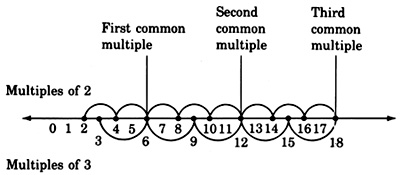# 1.4: The Least Common Multiple

$$\newcommand{\vecs}{\overset { \rightharpoonup} {\mathbf{#1}} }$$ $$\newcommand{\vecd}{\overset{-\!-\!\rightharpoonup}{\vphantom{a}\smash {#1}}}$$$$\newcommand{\id}{\mathrm{id}}$$ $$\newcommand{\Span}{\mathrm{span}}$$ $$\newcommand{\kernel}{\mathrm{null}\,}$$ $$\newcommand{\range}{\mathrm{range}\,}$$ $$\newcommand{\RealPart}{\mathrm{Re}}$$ $$\newcommand{\ImaginaryPart}{\mathrm{Im}}$$ $$\newcommand{\Argument}{\mathrm{Arg}}$$ $$\newcommand{\norm}{\| #1 \|}$$ $$\newcommand{\inner}{\langle #1, #2 \rangle}$$ $$\newcommand{\Span}{\mathrm{span}}$$ $$\newcommand{\id}{\mathrm{id}}$$ $$\newcommand{\Span}{\mathrm{span}}$$ $$\newcommand{\kernel}{\mathrm{null}\,}$$ $$\newcommand{\range}{\mathrm{range}\,}$$ $$\newcommand{\RealPart}{\mathrm{Re}}$$ $$\newcommand{\ImaginaryPart}{\mathrm{Im}}$$ $$\newcommand{\Argument}{\mathrm{Arg}}$$ $$\newcommand{\norm}{\| #1 \|}$$ $$\newcommand{\inner}{\langle #1, #2 \rangle}$$ $$\newcommand{\Span}{\mathrm{span}}$$$$\newcommand{\AA}{\unicode[.8,0]{x212B}}$$

## Overview

• Multiples
• Common Multiples
• The Least Common Multiple (LCM)
• Finding The Least Common Multiple

## Multiples

##### Definition: Multiples

When a whole number is multiplied by other whole numbers, with the exception of Multiples zero, the resulting products are called multiples of the given whole number.

 Multiples of 2 Multiples of 3 Multiples of 8 Multiples of 10 2·1=2 3·1=3 8·1=8 10·1=10 2·2=4 3·2=6 8·2=16 10·2=20 2·3=6 3·3=9 8·3=24 10·3=30 2·4=8 3·4=12 8·4=32 10·4=40 2·5=10 3·5=15 8·5=40 10·5=50 … … … …

### Common Multiples

There will be times when we are given two or more whole numbers and we will need to know if there are any multiples that are common to each of them. If there are, we will need to know what they are. For example, some of the multiples that are common to 2 and 3 are 6, 12, and 18.

### Sample Set A

##### Example $$\PageIndex{1}$$

We can visualize common multiples using the number line.Notice that the common multiples can be divided by both whole numbers.

### The Least Common Multiple (LCM)

Notice that in our number line visualization of common multiples (above) the first common multiple is also the smallest, or least common multiple, abbreviated by LCM.

##### Definition: Least Common Multiple (LCM)

The least common multiple, LCM, of two or more whole numbers is the smallest whole number that each of the given numbers will divide into without a remainder.

### Finding The Least Common Multiple

Finding the LCM

To find the LCM of two or more numbers,

1. Write the prime factorization of each number, using exponents on repeated factors.
2. Write each base that appears in each of the prime factorizations.
3. To each base, attach the largest exponent that appears on it in the prime factorizations.
4. The LCM is the product of the numbers found in step 3.

### Sample Set B

Find the LCM.

##### Example $$\PageIndex{2}$$

9 and 12

1. 9 = 3 · 3 = $$3^2$$
12 = 2
· 6 = 2 · 2 · 3 = $$2^2$$ · 3
2. The bases that appear in the prime factorizations are 2 and 3.
3. The largest exponents appearing on 2 and 3 in the prime factorizations are, respectively, 2 and 2 (or 22 from 12, and 32 from 9).
4. The LCM is the product of these numbers.

LCM =22  · 32 =4 · 9=36

Thus, 36 is the smallest number that both 9 and 12 divide into without remainders.

##### Example $$\PageIndex{3}$$

90 and 630

1. 90 = 2 · 45 = 2 · 3 · 15 = 2 · 3 · 3 · 5 = 2 · $$3^2$$ · 5
630 = 2 · 315 = 2 · 3 · 205 = 2 · 3 · 3 · 35 = 2 · 3 · 3 · 5 · 7 = 2 · $$3^2$$ · 5 · 7
2. The bases that appear in the prime factorizations are 2, 3, 5, and 7.
3. The largest exponents that appear on 2, 3, 5, and 7 are, respectively, 1, 2, 1, and 1.

$$2^1$$ from either 90 or 630
$$3^2$$ from either 90 or 630
$$5^1$$ from either 90 or 630
$$7^1$$ from 630
4. The LCM is the product of these numbers.

LCM =2 · 32  · 5 · 7 = 2 · 9 · 5 · 7 = 630

Thus, 630 is the smallest number that both 90 and 630 divide into with no remainders.

##### Example $$\PageIndex{4}$$

33, 110, and 484

1. 33 = 3 · 11
110 = 2 · 55 = 2 · 5 · 11
484 = 2 · 242 = 2 · 2 · 121 = 2 · 2 · 11 · 11 = $$2^2$$ · $$11^2$$
2. The bases that appear in the prime factorizations are 2, 3, 5, and 11.
3. The largest exponents that appear on 2, 3, 5, and 11 are, respectively, 2, 1, 1, and 2.

$$2^2$$ from 484
$$3^1$$ from 33
$$5^1$$ from 110
$$11^2$$ from 484
4. The LCM is the product of these numbers.

LCM = $$2^2$$ · 3 · 5 · $$11^2$$
= 4 · 3 · 5 · 121
= 7260

Thus, 7260 is the smallest number that 33, 110, and 484 divide into without remainders.

### Exercises:

For the following problems, find the least common multiple of given numbers.

##### Exercise $$\PageIndex{1}$$

8, 12

$$2^3$$ · $$3$$

8, 10

##### Exercise $$\PageIndex{3}$$

6, 12

$$2^2$$ · $$3$$

9, 18

5, 6

2 · 3 · 5

7, 9

##### Exercise $$\PageIndex{7}$$

28, 36

$$2^2$$ · $$3^2$$ · 7

24, 36

##### Exercise $$\PageIndex{9}$$

28, 42

$$2^2$$ · 3 · 7

20, 24

##### Exercise $$\PageIndex{11}$$

25, 30

$$2$$ · $$3$$ · $$5^2$$

24, 54

##### Exercise $$\PageIndex{13}$$

16, 24

$$2^4$$ · $$3$$

36, 48

##### Exercise $$\PageIndex{15}$$

15, 21

$$3$$ · $$5$$ · $$7$$

7, 11, 33

##### Exercise $$\PageIndex{17}$$

8, 10, 15

$$2^3$$ · 3 · 5

4, 5, 21

##### Exercise $$\PageIndex{19}$$

45, 63, 98

$$2$$ · $$3^2$$ · $$5$$ · $$7^2$$

15, 25, 40

##### Exercise $$\PageIndex{21}$$

12, 16, 20

$$2^4$$ · $$3$$ · $$5$$

12, 16, 24

##### Exercise $$\PageIndex{23}$$

12, 16, 24, 36

$$2^4$$ · $$3^2$$